Ex 12.2

Chapter 12 Class 6 Ratio and Proportion
Serial order wise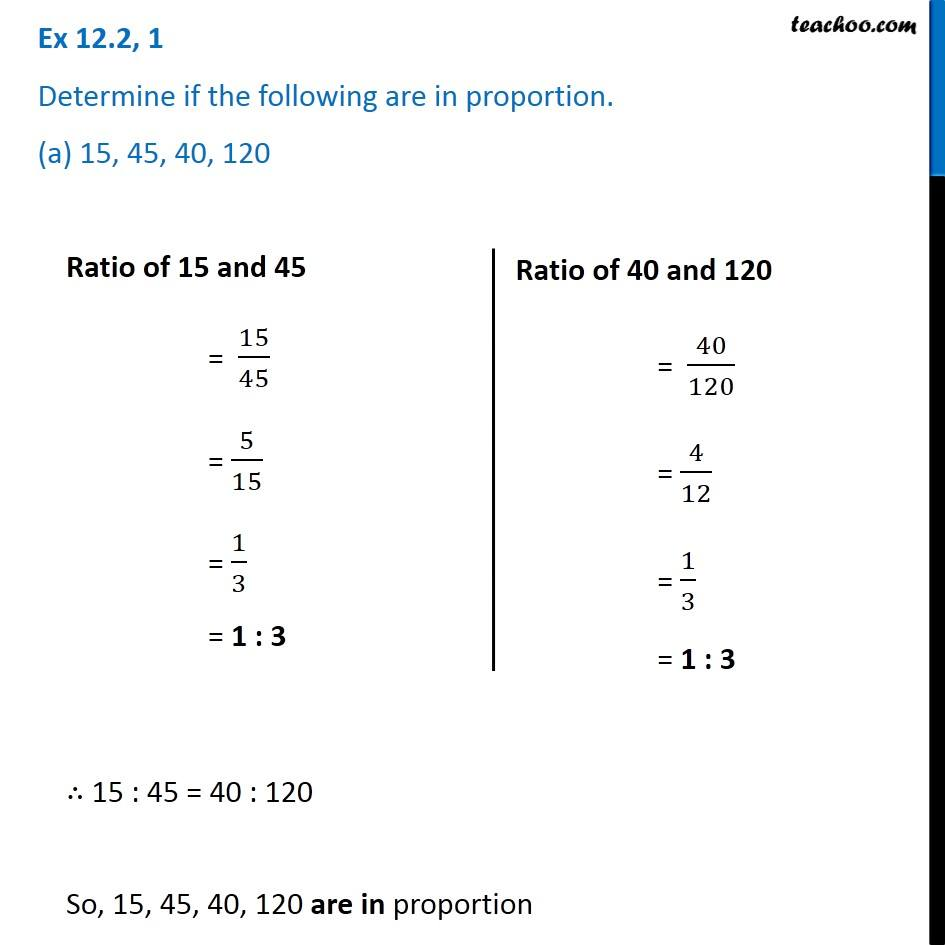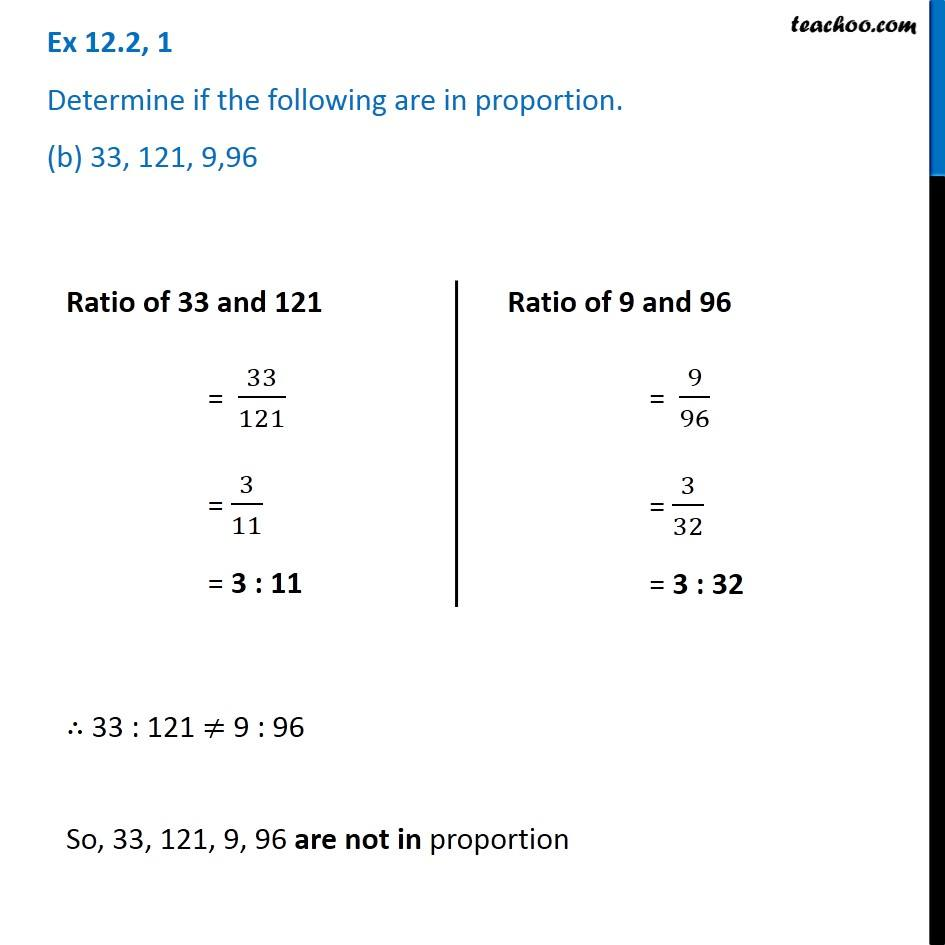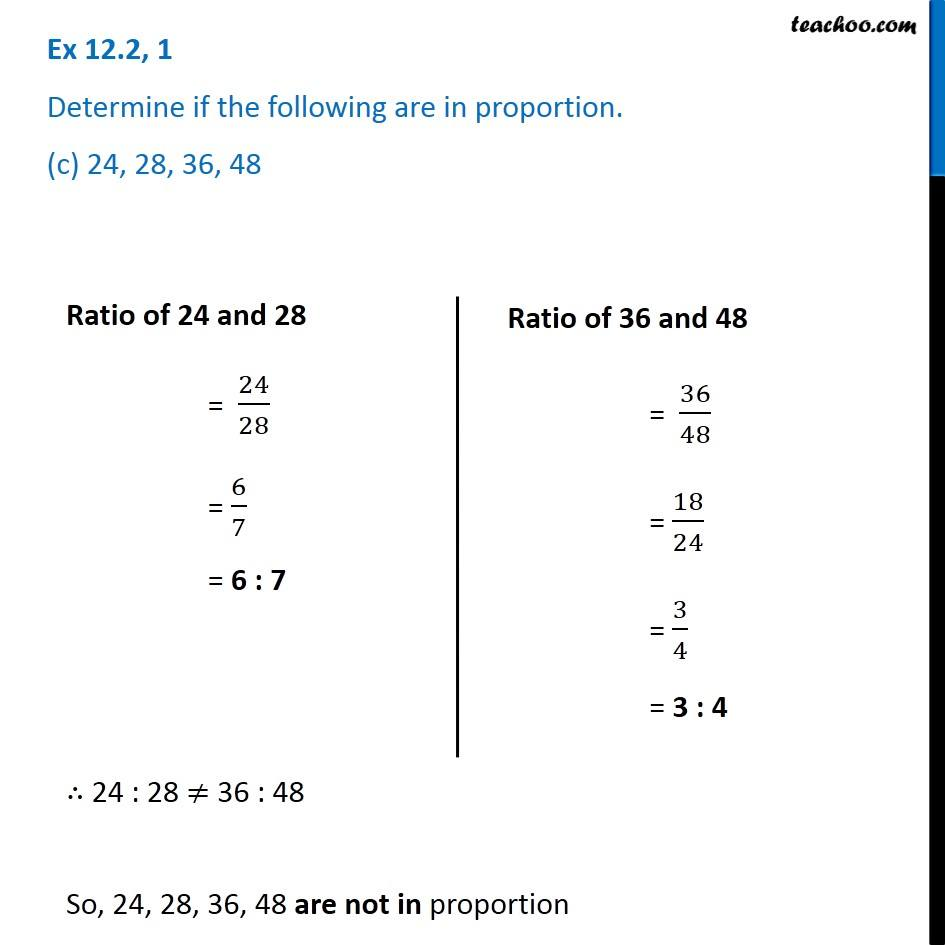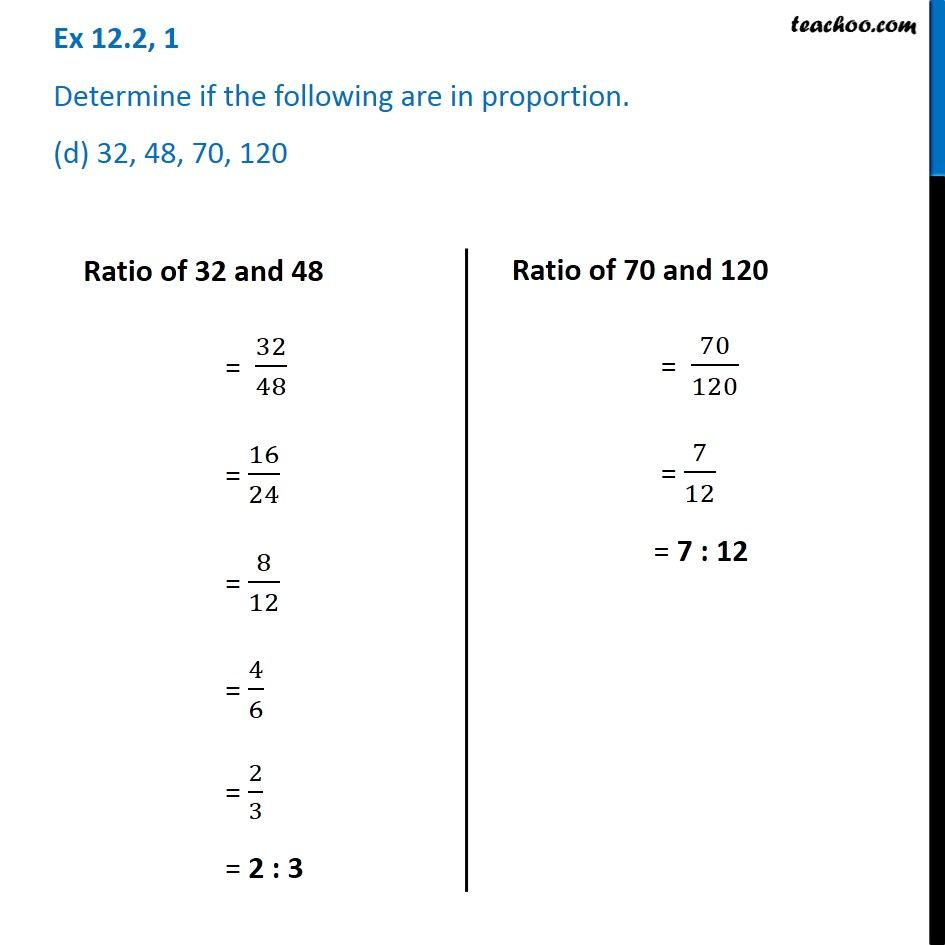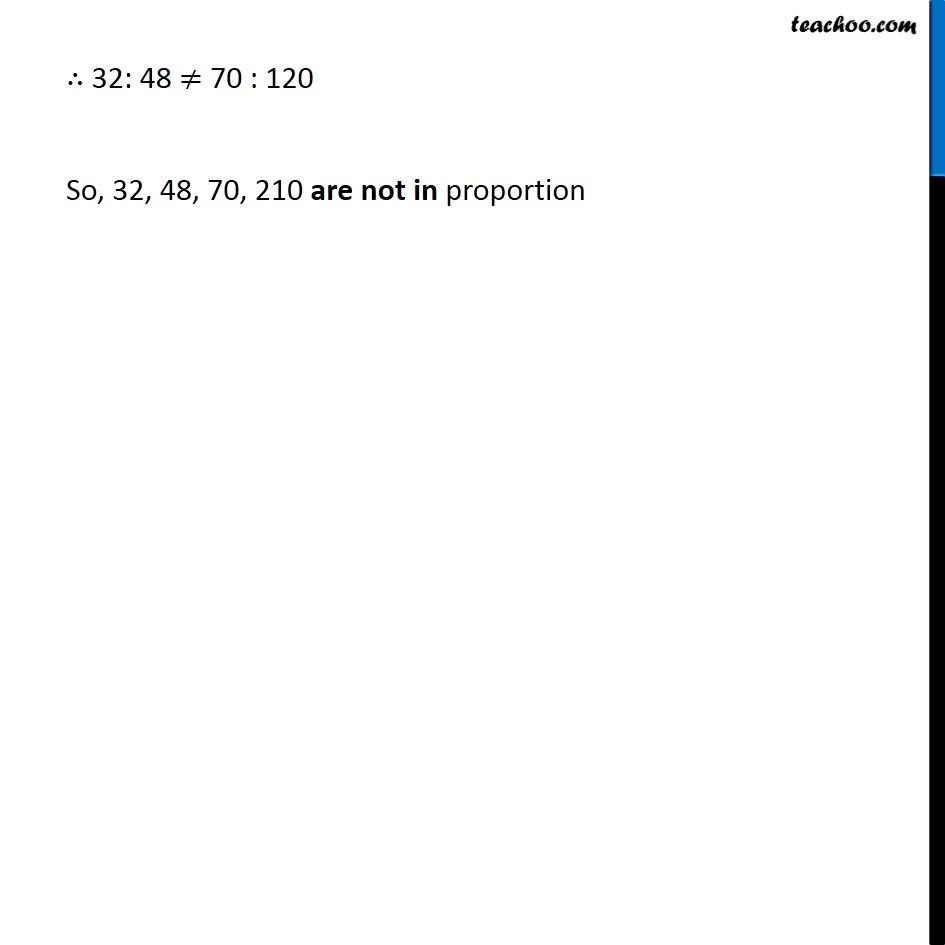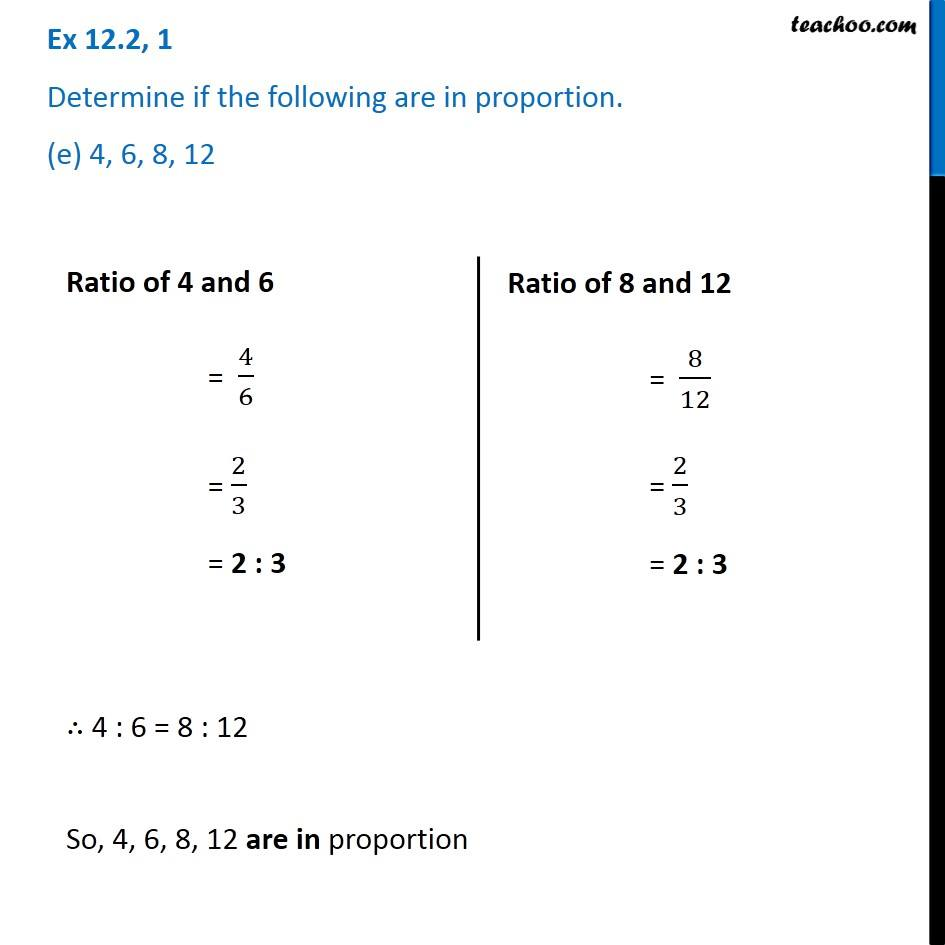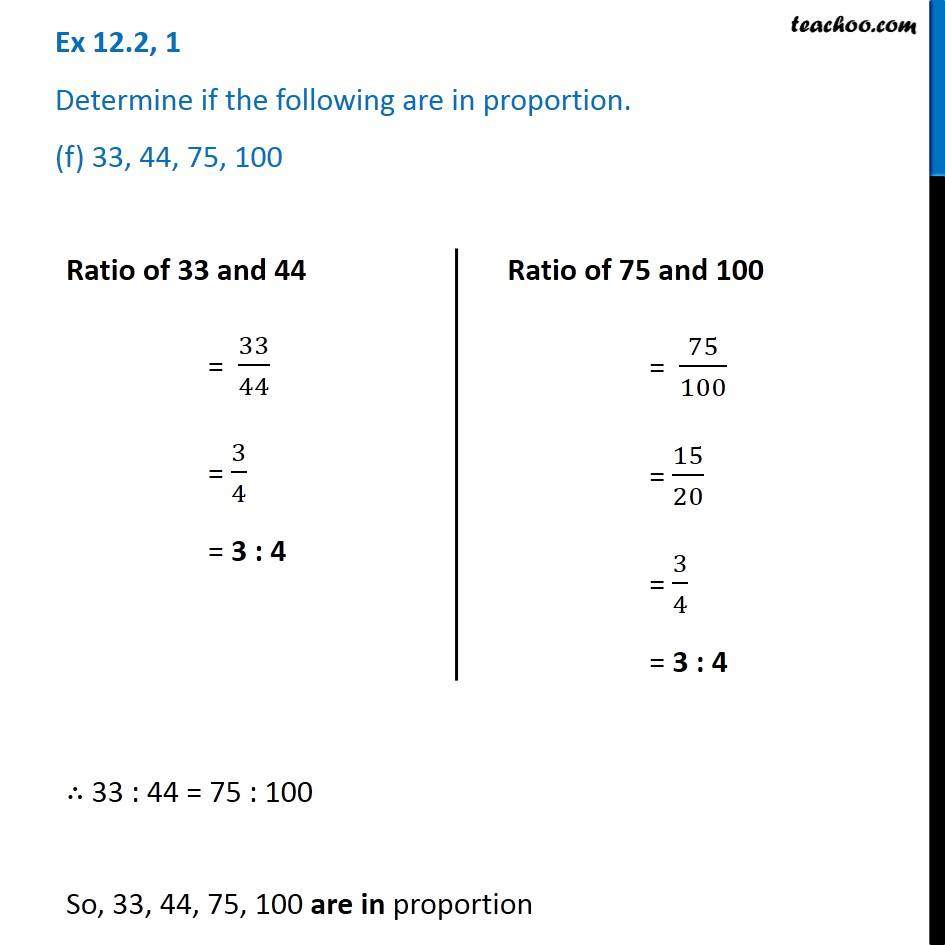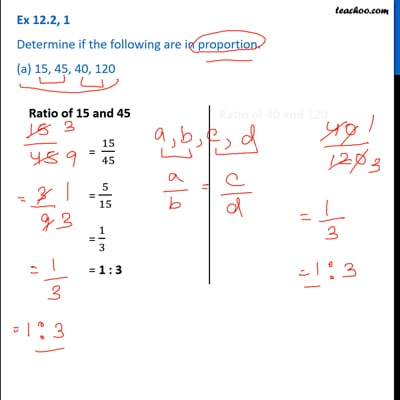This video is only available for Teachoo black users

Learn in your speed, with individual attention - Teachoo Maths 1-on-1 Class

### Transcript

Ex 12.2, 1 Determine if the following are in proportion. (a) 15, 45, 40, 120Ratio of 15 and 45 = 15/45 = 5/15 = 1/3 = 1 : 3 Ratio of 40 and 120 = 40/120 = 4/12 = 1/3 = 1 : 3 ∴ 15 : 45 = 40 : 120 So, 15, 45, 40, 120 are in proportion Ex 12.2, 1 Determine if the following are in proportion. (b) 33, 121, 9,96 Ratio of 33 and 121 = 33/121 = 3/11 = 3 : 11 Ratio of 9 and 96 = 9/96 = 3/32 = 3 : 32∴ 33 : 121 ≠ 9 : 96 So, 33, 121, 9, 96 are not in proportion Ex 12.2, 1 Determine if the following are in proportion. (c) 24, 28, 36, 48 Ex 12.2, 1 Determine if the following are in proportion. (d) 32, 48, 70, 120Ratio of 32 and 48 = 32/48 = 16/24 = 8/12 = 4/6 = 2/3 = 2 : 3 Ratio of 70 and 120 = 70/120 = 7/12 = 7 : 12 ∴ 32: 48 ≠ 70 : 120 So, 32, 48, 70, 210 are not in proportion Ex 12.2, 1 Determine if the following are in proportion. (e) 4, 6, 8, 12 ∴ 32: 48 ≠ 70 : 120 So, 32, 48, 70, 210 are not in proportion Ex 12.2, 1 Determine if the following are in proportion. (e) 4, 6, 8, 12 Ratio of 4 and 6 = 4/6 = 2/3 = 2 : 3 Ratio of 8 and 12 = 8/12 = 2/3 = 2 : 3 ∴ 4 : 6 = 8 : 12 So, 4, 6, 8, 12 are in proportion Ex 12.2, 1 Determine if the following are in proportion. (f) 33, 44, 75, 100Ratio of 33 and 44 = 33/44 = 3/4 = 3 : 4 Ratio of 75 and 100 = 75/100 = 15/20 = 3/4 = 3 : 4 ∴ 33 : 44 = 75 : 100 So, 33, 44, 75, 100 are in proportion